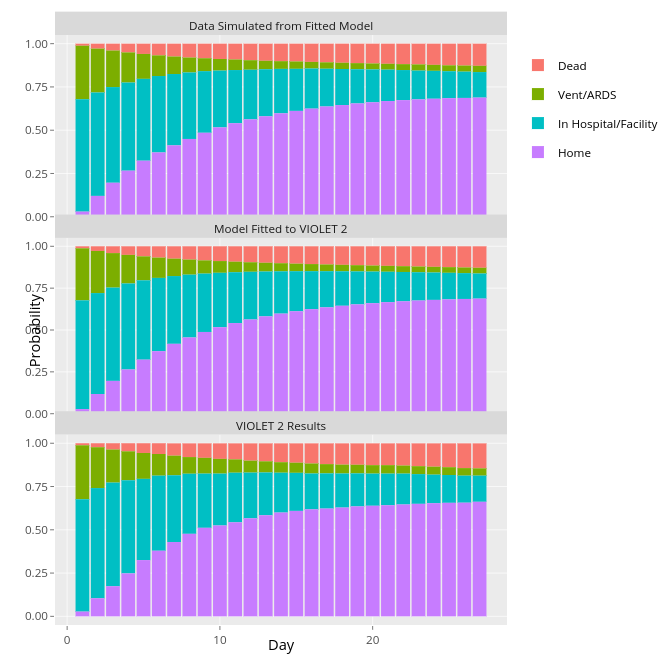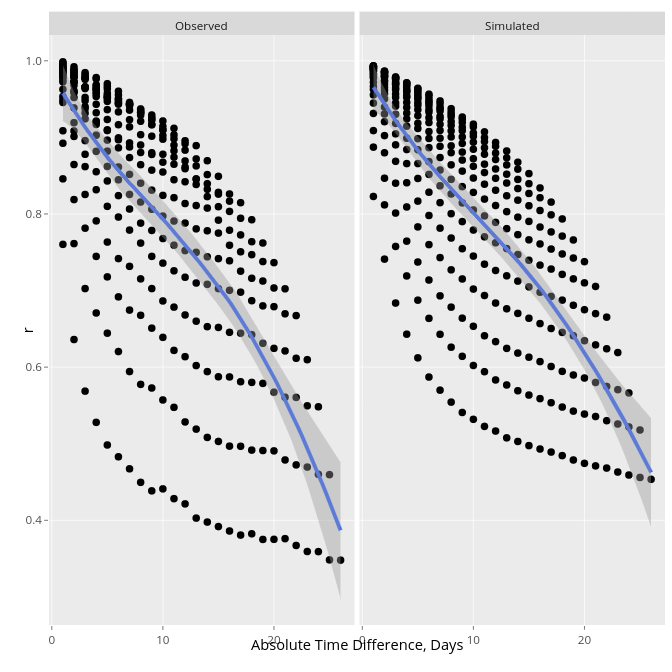CFE-CMStatistics 2021
2021-12-18

Univariate Proportional Odds Model

• $$\Pr(Y \geq y | X) = \mathrm{expit}(\alpha_{y} + X\beta)$$
• $$Y$$-transformation invariant, does not use $$Y$$ spacings
• Handles arbitrarily heavy ties or continuous $$Y$$, bi-modality, …
• Direct competitor of the linear model

PO Model, continued

• Wilcoxon-Mann-Whitney test: concordance probability $$c \approx \frac{OR^{0.66}}{1 + OR^{0.66}}$$ whether or not PO holds
• Kruskal-Wallis test: same; $$\beta$$ for $$X=j$$ vs. $$X=i$$ reflects $$c$$ for $$X$$ vs. $$Y$$ computed on observations with $$X \in \{i, j\}$$
• See fharrell.com/post/wpo

Extensions to Allow Non-PO

• Partial PO model
• Constrained partial PO model
• analogous to unequal variances in linear models
• Peterson & Harrell, 1990

Full Likelihood Extensions

• Random intercepts/slopes
• assumed correlation structures may be unrealistic
• can’t have absorbing states
• Markov models
• most flexible, fastest, easiest to program
• require unconditioning on previous $$Y$$ to get marginal distributions
• Other marginal models (Lee & Daniels, Schildcrout); direct $$\Pr($$state occupancy$$)$$

First-Order Discrete Time Markov Proportional Odds Model

• Current state depends only on covariates, previous state, gap
• Let measurement times be $$t_{1}, t_{2}, \dots, t_{m}$$, and the measurement for a patient at time $$t$$ be denoted $$Y(t)$$ $\Pr(Y(t_{i}) \geq y | X, Y(t_{i-1})) =$ $\mathrm{expit}(\alpha_{y} + X\beta + g(Y(t_{i-1}), t_{i}, t_{i} - t_{i-1}))$

Examples of Parametric Modeling Previous State in $$g$$:

• Linear in numeric codes for $$Y$$
• Single binary indicator for a specific state such as the lowest or highest one
• Discontinuous bi-linear relationship where there is a slope for in-hospital outcome severity, a separate slope for outpatient outcome severity, and an intercept jump at the transition from inpatient to outpatient (or vice versa).

Most Important Effects to Include

• Previous state
• Flexible function of time $$t$$ since randomization
• No time effect $$\sim$$ constant hazard rate
• Non-proportional odds effects for $$t$$
• Mix of events can change over time, e.g., early ventilator use, late death
• $$t \times$$ previous state interaction
• Example: Hospitalized patients more stable over time = increasing effect of previous state

Effects to Possibly Include, continued

• Flexible function of gap times (if gap times and absolute time are virtually collinear, one of these may be omitted)
• Interaction between previous state and gap time if gaps are very non-constant
• Interaction between time and treatment if treatment effect is delayed, etc.

Markov PO Model: A Unified Approach

• Time to terminating event
• transition probability = discrete hazard rate
• OR $$\approx$$ HR when time intervals small
• easily handles time-dependent covariates, left-truncation
• Recurrent binary events
• Recurrent binary events + a terminal event

Unified Approach, continued

• Competing risks
• death explicitly handled as a bad outcome
• easier to interpret than competing risk models
• Serial current status data
• events of different severities
• no need to judge whether an early heart attack is worse than a late death
• Missing data and interval-censored $$Y$$

Unified Approach, continued

• Standard longitudinal continuous $$Y$$
• Longitudinal continuous or ordinal $$Y$$ interrupted by clinical events
• Easily handles multiple absorbing states

Examples of Longitudinal Ordinal Outcomes

• censored at 3w: 000
• death at 2w: 01
• 0=at home 1=hospitalized 2=MI 3=dead
• hospitalized at 3w, rehosp at 7w, MI at 8w & stays in hosp, f/u ends at 10w: 0010001211

Examples, continued

• 0-6 QOL excellent–poor, 7=MI 8=stroke 9=dead
• QOL varies, not assessed in 3w but pt event free, stroke at 8w, death 9w: 12[0-6]334589
• MI status unknown at 7w: 12[0-6]334[5,7]89
• Can make first 200 levels be a continuous response variable and the remaining values represent clinical event overrides

Quality of Fit in a Critical Illness RCT

• VIOLET trial from the PETAL network, NHLBI
• Vitamin D in critically ill adults
• Daily assessments for 28d on 4-level ordinal $$Y$$: home, hospitalized, vent/ARDS, dead
• See here for details and codeSecond-Order Fit

• Add random effects: negligible (indicates conditional independence)
• VariogramUnconditioning on Previous States

• For equal time spacing:
$$\Pr(Y(t)=y | X) =$$
$$\sum_{j=1}^{k}\Pr(Y(t)=y | X, Y(t-1) = j) \times$$
$$\Pr(Y(t-1) = j | X)$$
• Use this recursively
• Yields a semiparametric unconditional (except for $$X$$) distribution of $$Y$$ at each $$t$$ (SOPs)
• soprobMarkovOrd* functions in the R Hmisc package make this easy for frequentist and Bayesian models

Estimands

• Transition odds ratios (original parameters)
• Prior state and covariate-specific transition probabilities
• Covariate-specific SOPs
• $$\Pr($$stroke in week 4 or death in or before week 4$$)$$; $$\Pr($$stroke and alive$$)$$
• Time in state $$Y=y$$ (like RMST)
• Time in states $$Y \geq y$$ (e.g., mean time unwell)
• Differences in mean time in state between treatments

Special Advantage of Bayesian Models and MCMC Posterior Sampling

• SOPs involve complex derived parameters for which frequentist CLs are very hard to derive
• Bayesian posterior distribution and uncertainty intervals derived from it are trivial to compute
• Example: 4,000 posterior draws from transition model’s basic parameters; compute 4,000 values of each derived parameter (SOP; mean time in state, etc.)

Software

• Frequentist: R VGAM package
• Bayesian: R rmsb package